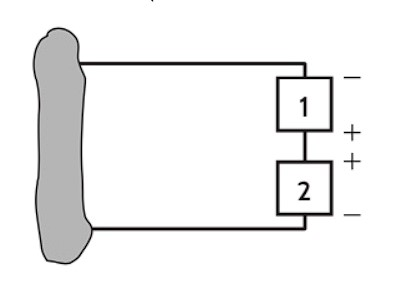Home / Expert Answers / Electrical Engineering / q6-in-nbsp-fig-p2-41-watts-how-much-power-is-absorbed-by-element-1-element-1-9-volts-el-pa614

# (Solved): (Q6) In Fig, P2 = -41 Watts. How much power is absorbed by element 1 ? (Element 1 = 9 Volts, El ...

(Q6) In Fig, P2 = -41 Watts. How much power is absorbed by element 1 ? (Element 1 = 9 Volts, Element 2 = 14 Volts)Notes on entering solution:

Enter your solution to the nearest hundredth of a Watt

Do not include units in you answer

ex. 5W is entered as 5.00